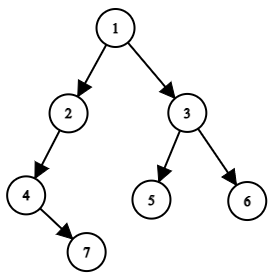Problem title
Difficulty
Avg time to solve

Data Structure Supporting Insert Delete And GetRandom
Easy
15 mins
Permutation In String
Hard
20 mins
Maximum number in K swaps
Hard
15 mins
Check if the door is open or closed
Easy
10 mins
Minimum Sum Subarray
Easy
15 mins
Easy
10 mins
Matrix Chain Multiplication
Easy
--
Largest Prime Factor
Easy
15 mins
Palindrome Partitioning ll
Moderate
15 mins
Sub-Matrix with Sum Zero
Moderate
35 mins3

# Spiral Order Traversal of a Binary Tree

Difficulty: EASY
Avg. time to solve
20 min
Success Rate
75%

Problem Statement

#### You have been given a binary tree of 'N' nodes. Print the Spiral Order traversal of this binary tree.

##### For example
``````For the given binary tree [1, 2, 3, -1, -1, 4, 5, -1, -1, -1, -1]
1
/ \
2   3
/ \
4   5

Output: 1 3 2 4 5
``````
##### Input Format
``````The only line of input contains elements of the tree in the level order form. The line consists of values of nodes separated by a single space. In case a node is null, we take -1 in its place.
````````````For example, the input for the tree depicted in the above image would be :

1
2 3
4 -1 5 6
-1 7 -1 -1 -1 -1
-1 -1
``````
##### Explanation
``````Level 1 :
The root node of the tree is 1

Level 2 :
Left child of 1 = 2
Right child of 1 = 3

Level 3 :
Left child of 2 = 4
Right child of 2 = null (-1)
Left child of 3 = 5
Right child of 3 = 6

Level 4 :
Left child of 4 = null (-1)
Right child of 4 = 7
Left child of 5 = null (-1)
Right child of 5 = null (-1)
Left child of 6 = null (-1)
Right child of 6 = null (-1)

Level 5 :
Left child of 7 = null (-1)
Right child of 7 = null (-1)
``````
##### Note
``````The above format was just to provide clarity on how the input is formed for a given tree.
The sequence will be put together in a single line separated by a single space. Hence, for the above-depicted tree, the input will be given as:

1 2 3 4 -1 5 6 -1 7 -1 -1 -1 -1 -1 -1
``````
##### Output Format:
``````Print 'N' single space-separated integers representing the spiral order traversal of the binary tree.
``````
##### Note
``````You do not need to print anything, it has already been taken care of. Just implement the given function and return the list of elements containing the spiral order of the given input tree.
``````
##### Constraints
``````0 <= N <= 10 ^ 4

Where 'N' is the total number of nodes in the binary tree

Time Limit: 1 sec
``````
##### Sample Input 1
``````1 2 3 4 -1 5 6 -1 7 -1 -1 -1 -1 -1 -1
``````
##### Sample Output 1:
``````1 3 2 4 5 6 7
``````
##### Explanation of Sample Input 1:``````From the above-depicted representation of the input tree,

Level-0: 1(taken in the left to right fashion)
Level-1: 3 2(taken in the right to left fashion)
Level-2: 4 5 6(taken in the left to right fashion)
Level-3: 7(taken in the right to left fashion)

When taken all the sequences linearly from levels 0 to 3, we get [1, 3, 2, 4, 5, 6, 7] and hence the desired output.
``````
##### Sample Input 2
``````1 2 3 -1 4 5 6 7 8 -1 -1 -1 -1 -1 -1 -1 -1
``````
##### Sample Output 2
``````1 3 2 4 5 6 8 7
``````Console